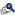On the Problem of Negative Variance Component Estimates in Mixed ModelsView Presentation *Walter Stroup, University of Nebraska, Lincoln  Keywords: Negative variance component estimates in mixed model analysis raise two issues: 1) interpretation when the variance component is a primary focus of inference; 2) the impact of negative estimates on inference involving treatment effects. Littell and Stroup (2002) discussed fixed effect inference in two-way unbalanced linear mixed models with negative variance estimates, but the subject remains controversial and widely misunderstood. Recently, SAS® introduced the GLIMMIX procedure, making mixed model procedures for non-normal data widely accessible. As a result, questions about best practices when one encounters negative variance components now include non-normal as well as Gaussian data. This presentation reviews what we know about fixed effect inference in the presence of negative variance component estimates with Gaussian mixed models, focusing on models typically used in pharmaceutical research. We then consider generalized linear mixed models, emphasizing binomial and count data. While some guidelines for Gaussian data also apply to the generalized case, non-normal data introduce additional considerations. For example, overdispersion and variance component estimation are often confounded issues. Examples will illustrate problems that can arise with generalized linear mixed models. Where possible, suggested best practices are outlined. Where not possible, areas needing further research are suggested.